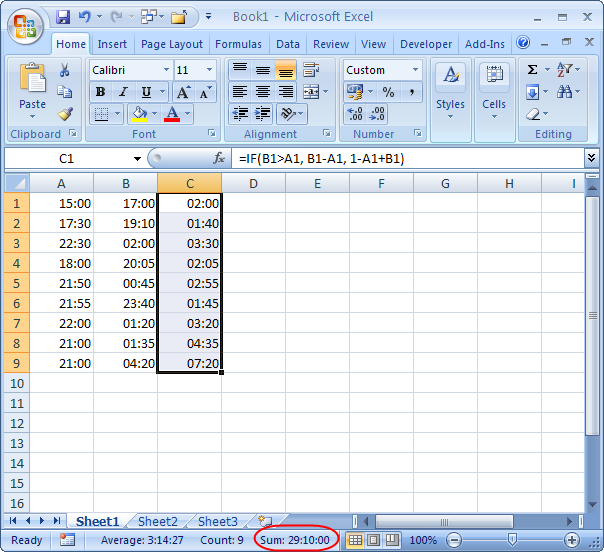Sometimes, when I’m working on a project, I need to keep stopping and starting. Although I’ve used various stopwatch programs in the past, these days I often just write down the start and end times of each batch of work and then work out the total later.

To get a total count of hours, I simply type the start and end times into a new Microsoft Excel worksheet in Columns A and B. If the values are entered as times, their differences are also times. I use a simple formula in Column C to calculate the difference between the two times, coping with the case where I have worked over midnight.The formula can be interpreted as “if the value in B is greater than the value in A then both dates are in the same day, so take their difference; otherwise add the time from A to midnight to the time in B”. Note that time values in Excel range from 0 to 1, so 1-A1 gives the time value from A1 to midnight.

Dragging a selection across all the calculated times gives their total in the status bar at the bottom of the window. So, the example project shown in the screenshot took 29 hours 10 minutes to complete.